INSTITUTE FOR THEORETICAL AND MATHEMATICAL PHYSICS

Lomonosov Moscow State University# Scientific Members

•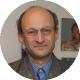Director of ITMP

Research interests of Arkady Tseytlin include quantum field theory and quantum gravity, superstring theory, conformal theories and AdS/CFT correspondence. He obtained several key results in superstring theory and field theory. In particular, he developed the sigma model approach to string theory, discovered the fundamental role of Born-Infeld action as the open string effective action, developed the method of constructing composite solitonic solutions in supergravity describing supersymmetric bound states of branes, contributed to investigations of D-branes that led to AdS/CFT duality, constructed the action of superstings in AdS5 x S5 space and made substantial contributions to the integrability-based approach to gauge-string duality.

•Senior Researcher

The main focus of Konstantin’s research is the AdS/CFT correspondence for QFTs with higher symmetries such as 2d CFT and HS gravity. He and collaborators pioneered studies of the frame-like formulation for general free and interacting massless AdS fields, built mixed-symmetry conserved currents along with their duals, proposed higher-spin extensions of the Jackiw-Teitelboim gravity. More recent results include the combinatorial representation for minimal CFT with W symmetry, the semiclassical AdS/CFT correspondence between higher-point conformal blocks and geodesic trees, large-с torus CFT blocks and their duals.

•Senior Researcher

Scientific interests include quantum field theory, quantum gravity and cosmology, and their applications in the physics of the early and modern universe.

•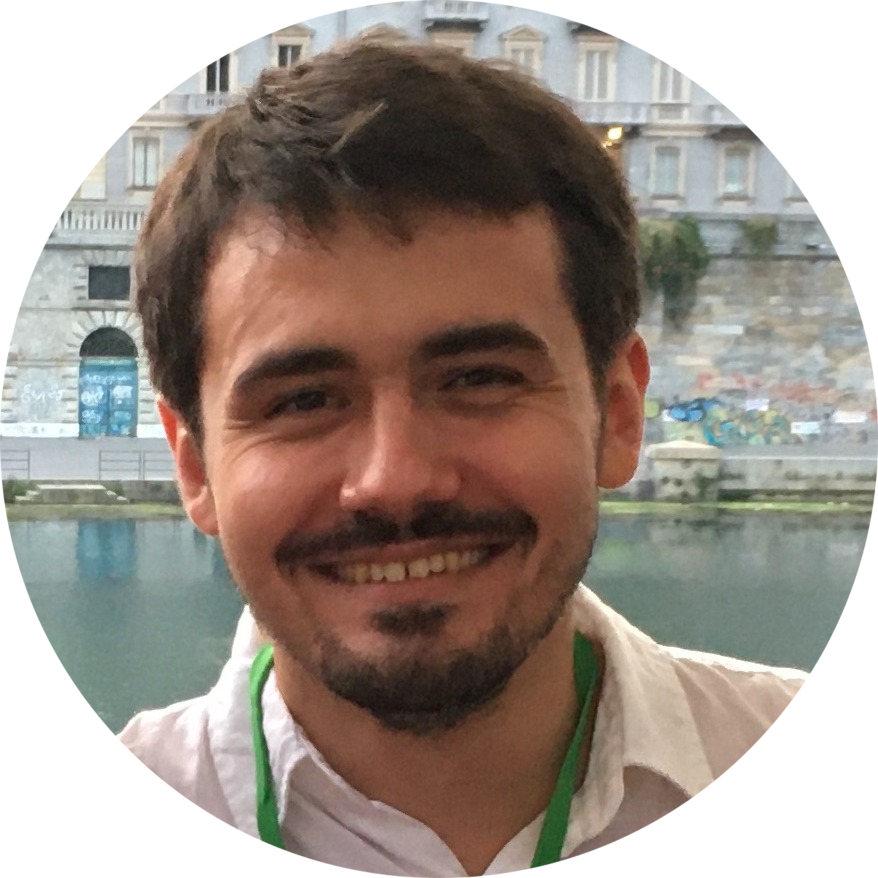Senior Researcher

My scientific interests are related to the application of geometric methods in theoretical/mathematical physics. Results are in the theory of flag manifold sigma models, integrable sigma models, as well as in the construction of Ricci-flat metrics on non-compact Kähler manifolds and in the study of mathematical aspects of Nekrasov-Shatashvili theory. In particular, I showed that certain spin chains with SU(N) symmetry are described, in a continuum limit, by flag manifold sigma models, which later on led to a formulation of the so-called generalized Haldane conjectures for such systems by I.Affleck et.al. In the theory of integrable models I formulated a conjecture about the integrability of certain models with non-symmetric target spaces (such as flag manifolds). It was subsequently found that such models naturally fit in a general framework developed by C.Costello and M.Yamazaki and that, in fact, there is a wider class of integrable models with quiver (super)variety phase spaces, which from a physics perspective are equivalent to generalized Gross-Neveu models with Bose- and Fermi- fields.

•Senior Researcher

Scientific interests of Andrei Zotov are mainly related to the theory of integrable systems, its methods and numerous applications. A series of his papers is devoted to interrelations (dualities) between different type models. For example, an interesting correspondence was observed between the classical many-body systems and quantum spin chains. Among other issues, these type dualities allow to predict some properties for solutions of the Painleve equations, the Knizhnik-Zamolodchikov equations as well as many other important equations in theoretical and mathematical physics.

•Deputy Director

Scientific interests of Maxim Grigoriev involve mathematical foundations of gauge systems (constrained dynamics and symmetries, Batalin-Vilkovisky quantization) higher-spin gauge theories and holography, superstring sigma models and noncommutative theories. He proposed the so-called parent formulation of general gauge theories which systematically unifies Batalin-Vilkovisky and Hamiltonian BRST approaches into a unique formalism which has the structure of (generalized) Alexandrov-Kontsevich-Schwartz-Zaboronsky (AKSZ) sigma-model, which is especially useful in the context of diffeomorphism-invariant theories and higher spin holography.

•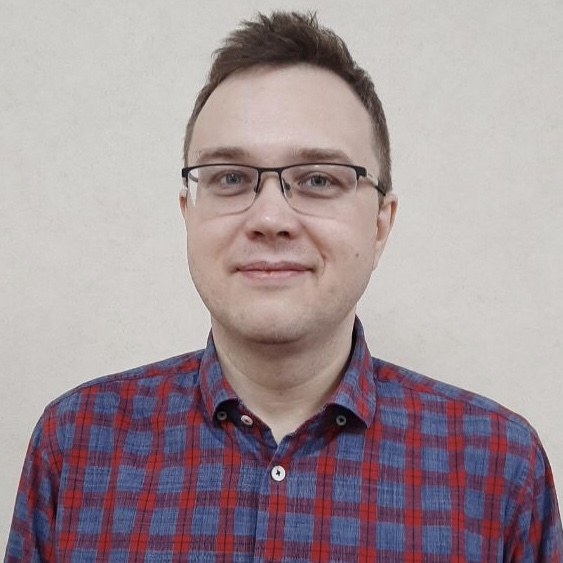Deputy Director

Dr. Levkov is a research fellow of ITMP and Institute for Nuclear Research RAS. His scientific interests are shared between cosmology of ultra-light dark matter, black hole physics, instantons and semiclassical description of nonperturbative transitions in quantum field theory. He and his collaborators for the first time computed the probability of baryon number violation in electroweak particle collisions at colliders, were first to describe kinetic Bose-Einstein condensation of light dark matter due to gravitational interactions.

•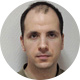Research Fellow

Scientific interests of Sergey Mironov involve the mathematical aspects of modern cosmology and gravity, including theories with higher derivatives, the AGT conjecture between gauge and conformal theories, topological field theories, especially knot theory, as well as their connection with integrable systems. In collaboration with his collegues he derived a number of formulas for universal parts of the triple functions for the W algebra. Another important result is the discovery of no-go theorems for stable classical solutions in theories of gravity with scalar fields of quite general form.

•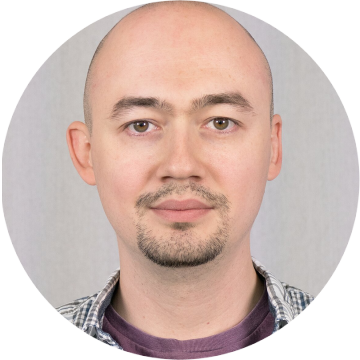Research Fellow

Research efforts are mainly focused at investigation of M-theory by field-theoretical methods with further applications to a description of conformal field theories and their RG flows in the context of gauge/gravity duality. The main research tools to address these problems are provided by the so-called exceptional field theory, that is a U-duality covariant formulation of supergravity developed by Edvard and collaborators a decade ago. Based on this approach are more recent results, that include a full T-covariant formulation of the T-duality orbit of the NS5B-brane (including exotic states); an algorithm to deform 11D supergravity solutions generalizing Yang-Baxter deformations; discovery of a class of tri-vector deformations dual to non-supersymmetric exactly marginal deformations of SCFT's; formulation of a generalization of 11D supergravity similar to generalized 10D supergravity related to a reformulation of the kappa-symmetry constraint of a Green-Schwarz superstring.

•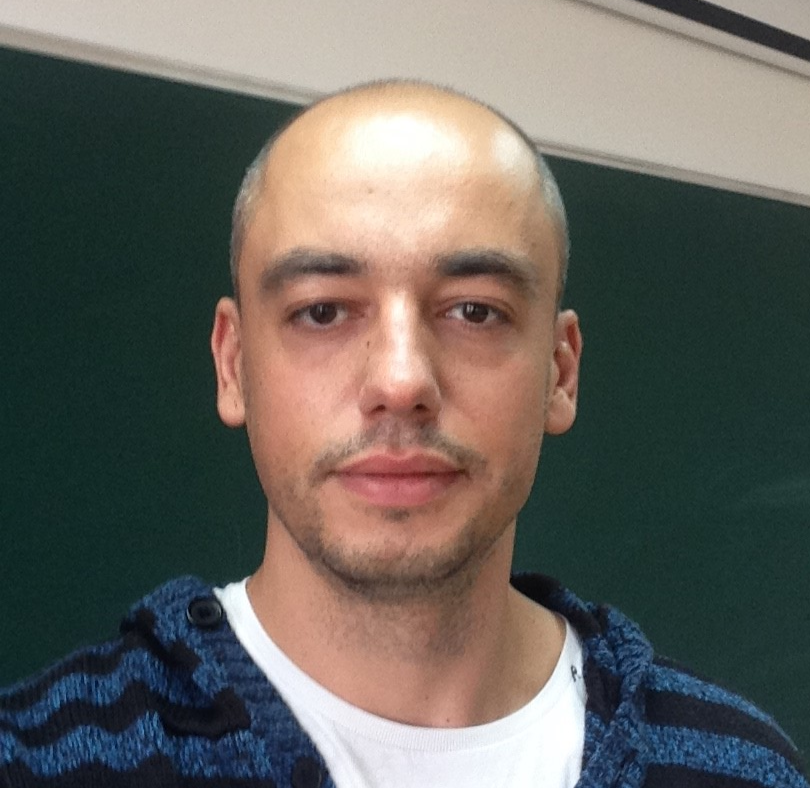Senior Researcher

I am mainly interested in constructing and analyzing interacting theories of higher-spin gauge fields as well as in other closely related problems of classical and quantum field theories. In particular, together with colleagues we were first to derive quartic vertices in higher spin theories from AdS/CFT correspondence and studied their locality, constructed chiral higher-spin theories employing the light-cone gauge approach and established the relation of the latter theories to self-dual Yang Mills theory and sigma models, as well as computed simplest quantum corrections in higher-spin theories.

•Research Fellow

Mathematical physics is breathtakingly beautiful – it is an interplay between physics and pure mathematics with a tremendous power to model reality, as well as to create stunning worlds of its own. My research interests are at the interface of symplectic geometry, representation theory, algebraic topology, gauge theories, quantum field theories and string theory.